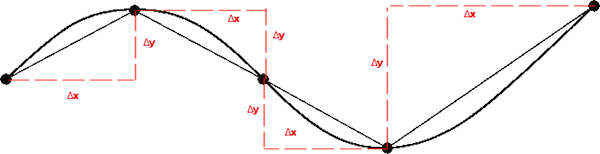# Arc Length

## Definition

The arc length, $$s$$ along the graph of a parametric function $$(x,y) = \left(x(t),y(t)\right)$$ from $$t=a$$ to $$t=b$$ is given by:

$s = \int_a^b \sqrt{\left( \frac{dx}{dt} \right)^2+\left( \frac{dy}{dt} \right )^2 }\, dt$

We can see why by examining a linear approximation of the arclength:$\Delta s^2 = \Delta x^2 + \Delta y^2$ by the Pythagorean Theorem, which means that for very small $\Delta s$, $ds \approx \Delta s = \sqrt{\Delta x^2 + \Delta y^2}$.

Also, notice that in the the case where $x(t)=t$, we have $(x,y) = (t,y(t))$, and the arc length becomes:

$s = \int_a^b \sqrt{1+\left( \frac{dy}{dt} \right )^2 }\, dt$

which we can use for non-parametrized functions.

## Technique

### Show that the arc length of a semi-circle of radius 1 is $\pi$.

Let $(x,y)=(\cos \theta, \sin \theta)$, which describes the unit-circle. Then the arc length around the the semi-circle is given by:

$s = \int_0^\pi \sqrt{\left(\sin \theta\right)^2 + \left(- \cos \theta\right)^2}\, d\theta$

But $sin^2 \theta + \cos^2 \theta = 1$ by the Pythagorean Identity, so $s = \int_0^\pi\, d\theta = \pi. \, _\square$

## Application and Extensions

### Gabriel's Horn

Consider the surface area and volume of of the solid formed by rotating the region bounded by the $x$-axis, $y=1$, and $y = \frac{1}{x}$, around the $x$-axis. This solid is called Gabriel's Horn.#### Volume

The volume of the solid of revolution can be found using the disk method:

$V = \pi \int_0^a \frac{dx}{x^2} = \pi \left( 1 - \frac{1}{a} \right)$

Now consider what happens as we allow $a$ to approach infinity:

$\displaystyle \lim_{a \to \infty} \pi \left( 1 - \frac{1}{a} \right) = \pi$

#### Surface Area

The surface area of a solid of revolution is given by the formula

$S = 2\pi \int_a^b r(x)\sqrt{1+\left( \frac{dy}{dx} \right )^2 } \, dx$

In this case, since $\frac{d}{dx}\left(\frac{1}{x}\right) = -\frac{1}{x^2}$ that gives us:

\begin{aligned} S &= 2\pi \int_1^a \frac{1}{x} \sqrt{1+\left( -\frac{1}{x^2} \right )^2 } \, dx \\ &= 2\pi \int_1^a \frac{1}{x} \sqrt{1+ \frac{1}{x^4} } \, dx \end{aligned}

This integral is hard to evaluate, but since in our interval $\sqrt{1+ \frac{1}{x^4}} \geq 1$ and $\frac{1}{x} > 0$:

$2\pi \int_1^a \frac{1}{x} \sqrt{1+ \frac{1}{x^4} } \, dx \geq 2\pi \int_1^a \frac{1}{x} \, dx$

It follows that:

\begin{aligned} S &\geq 2\pi \int_1^a \frac{1}{x} \, dx \\ S &\geq 2\pi \ln a \end{aligned}

But $\displaystyle \lim_{a \to \infty} 2\pi \ln a = \infty$, which means that Gabriel's Horn has infinite surface area, but a volume of only $\pi$!Note by Arron Kau
7 years, 4 months ago

This discussion board is a place to discuss our Daily Challenges and the math and science related to those challenges. Explanations are more than just a solution — they should explain the steps and thinking strategies that you used to obtain the solution. Comments should further the discussion of math and science.

When posting on Brilliant:

• Use the emojis to react to an explanation, whether you're congratulating a job well done , or just really confused .
• Ask specific questions about the challenge or the steps in somebody's explanation. Well-posed questions can add a lot to the discussion, but posting "I don't understand!" doesn't help anyone.
• Try to contribute something new to the discussion, whether it is an extension, generalization or other idea related to the challenge.

MarkdownAppears as
*italics* or _italics_ italics
**bold** or __bold__ bold
- bulleted- list
• bulleted
• list
1. numbered2. list
1. numbered
2. list
Note: you must add a full line of space before and after lists for them to show up correctly
paragraph 1paragraph 2

paragraph 1

paragraph 2

[example link](https://brilliant.org)example link
> This is a quote
This is a quote
    # I indented these lines
# 4 spaces, and now they show
# up as a code block.

print "hello world"
# I indented these lines
# 4 spaces, and now they show
# up as a code block.

print "hello world"
MathAppears as
Remember to wrap math in $$ ... $$ or $ ... $ to ensure proper formatting.
2 \times 3 $2 \times 3$
2^{34} $2^{34}$
a_{i-1} $a_{i-1}$
\frac{2}{3} $\frac{2}{3}$
\sqrt{2} $\sqrt{2}$
\sum_{i=1}^3 $\sum_{i=1}^3$
\sin \theta $\sin \theta$
\boxed{123} $\boxed{123}$